Environmental Benefit Calculator Technical notes

This calculator was developed for the Little Stringybark Creek project by:
• Christopher J Walsh, University of Melbourne (maintainer: cwalsh@unimelb.edu.au);
• Tim D Fletcher, Monash University;
• Darren Bos, University of Melbourne;
• Sharyn RossRakesh, Melbourne Water;
• Veronika Nemes, Claire Edwards and Andrew O'Keefe, Department of Sustainability and Environment, Victoria.
• with subsequent improvements to the underlying code by Rhys Eddy of Enviss P/L
This page gives the full technical details of the revised EB calculator that was used for the Stormwater Fund, beginning 15 Feb 2010.
Technical details of the original (now non-functional) EB calculator (used for the Stormwater Tender 2008-2009) can be found here.

The pollutant removal in EB calculator is derived from research undertaken at the Facility for Advancing Water Biofiltration (FAWB: see www.monash.edu.au/fawb). We particularly wish to thank Dr. Belinda Hatt and Assoc. Prof. Ana Deletic for their contribution. The infiltration modelling was assisted by advice and calibration from Dale Browne (PhD student, Department of Civil Engineering, Monash University)
It uses RStudio Shiny, a package that permits a web-based applications using the mathematical and statistical program R.

The calculator uses two primary models written as R functions: tankmodel and gardenmodel, both in the file modelfunctions.R . Running these in R allows more flexibility: other rainfall records can be used, uses of tank water other than the calculator's limited options can be modelled (the 'other' argument in tankmodel), other soil profiles can be used (using the functions filter.profile to produce a data frame that is used for the filtr.prfile argument of gardenmodel).
More complex treatment trains can be calculated in R as well, using the functions tank.as.garden.inflow and garden.as.tank.inflow. (see this page for more information.)

Overview of the differences between the old and new EB calculators

• The new web calculator has been somewhat simplified, with fewer options (e.g consideration of unconnected surfaces, or the presence of septic tanks are no longer included): however, the new calculator gives the option of tanks with leaks for passive irrigation (which can substantially increase environmental benefit with little loss of yield from the tank)
• The old raingarden model assumed a uniform infiltration rate for surrounding soils. The new model assumes a variable soil profile closer to that observed in systems already built in Mt Evelyn. The infiltration capacity of the underlying soil = 0.005 mm/h, while that of the surface 30 cm is assumed to be 2.5 mm/h
• The old EB index was based on three subindices: a) reduction in flow frequency, b) reduction in nitrogen export, and c) volume of water consumed. The new EB index is based on four sub indices that are of more direct ecological relevance to streams: a) reduction in flow frequency (as before), b) equivalence between the pre-urban volume of subsurface flows and filtered flows from rain-gardens, c) median concentrations of 3 pollutants in filtered flows from rain-garden (N, P, TSS), and d) reduction in total volume of water exported (can be used or lost through evapo-transpiration).
• The old raingarden model assumed an erroneously high evapotranspiration (ET) loss (to correct for the overly conservative soil profile assumption). The new model assumes ET loss from a wet, vegetated raingarden of x sq m is equivalent to the theoretical potential ET loss from x sq m (using Bureau of Meteorology PET estimates). Unvegetated systems are assumed to have no ET loss. However, if an unlined raingarden is within 3 m of at least one large tree, the effective area available for ET loss is assumed to be equal to half the canopy area of the tree(s) (+ area of raingarden, if vegetated).

The calculator uses the rainfall record from Croydon, which is a few km west of Mt Evelyn. So, the results produced will be applicable for most of the eastern suburbs of Melbourne, and any other area with similar climate (temperate, mean annual rainfall of ~900 mm), and with thick, clayey soils

The caculator uses 3 years of rainfall data:
an average year, 1965 (actually Apr 1965 - Mar 1966), 956 mm;
a dry year, 1967, 661 mm;
a wet year, 1970, 1085 mm.
The old tankmodel calculated EB indices using the mean value for all three years, and the old gardenmodel used just data from 1965. In the new version, both the raingarden and tank models calculate EB indices using just 1965 data, although the web calculator uses the wet and dry year for graphical comparisons. The new calculator now gives the option of trying two other rainfall series (but these only use a single (average) year for now.

Still not enough detail for you? Read on!

To estimate tank water usage and overflow frequency, the R function tankmodel is used. This function requires input of the following variables (some of which EBI calculator requires the user to enter, and others that EBI calculator assumes values for).

1. Daily runoff or rainfall data. EB calculator uses daily runoff data for Croydon over three years that were chosen from the available record (6 minute records) from that site for which there was no missing data, and no accumulated data > 1 day in duration. The three years chosen represent a year with average rainfall (1965 &ndash actually 1 Apr 1965 &ndash 31 Mar 1966, with 950 mm of rain), a dry year (1967 with 660 mm), and a wet year (1970, with 1085 mm). Runoff was derived from hourly data (while none of the chose years had missing data, 1967 and 1970 each had nine blocks for which rainfall was aggregated, ranging from 2 to 24 hours &ndash for all but 3 of these blocks, the aggregated data was distributed across the blocks in proportion to rain that was recorded at the Melbourne station over the same block. For the remaining 3 blocks for which Melbourne also lacked data (all < 8 h), the aggregated rainfall was distributed evenly across the block. Runoff was estimated from the hourly data by assuming that the first 1 mm in a rain event was lost to evaporation (and therefore did not become runoff), and that a rain event was defined as any record of rain that followed at least one hour of zero recorded rain. This calculation of hourly runoff for the Croydon record was calculated using the function compile.inflow.R, and then converted to daily runoff. EB indices are calculated using 1965 data, but all three years are used for graphical comparisons.
2. Tank volume, and the volume of water in the tank at the beginning of the simulation. EB calculator uses the value entered for tank volume in the second tab, and assumes that the tank was 50% full at the start of the simulation.
3. Catchment area for the tank. EB calculator uses the roof area values entered in the 'Your tank' tab for this variable.
4. Initial loss of rainfall before it becomes runoff. EB calculator sets this to zero, because initial loss has been estimated already in the runoff input vector.
5. First flush volume. EB calculator requires this as an input. There are many types of first flush diverters that divert varying amounts of water from the tank before allowing runoff to enter. A critical assumption for EBI calculator is that any diverted first flush water either overflows to the modelled raingarden, or if there is no raingarden to the property's normal garden. If first flush water is diverted to a stormwater drain, then the estimated benefit to the stream should be considered zero.
6. Number of people living in the house. EB calculator uses the number of people entered in the first tab. This is used to calculate consumption patterns as below.

Water usage volumes are assumed as follows after Wilkenfield and associates (2006. Water saving requirements for new residential buildings in Victoria: options for flexible compliance. Melbourne: Department of Sustainability and Environment.). When each use is selected on the second tab the following values are used.

1. Toilet. EBI calculator assumes toilets use 18.9 L per person per day.
2. Washing machine. EBI calculator assumes washing machines use 35.31 L per day for the first person and 23.54 L for each additional person.
3. Hot water systems. EBI calculator assumes that hot water systems use 46.9 L per person per day (calculated from Wilkenfield's estimate of 61.9 minus half the washing machine use).
4. Garden area. EBI calculator uses the value entered in the second tab
5. Annual outdoor irrigation and monthly distribution. EBI calculator assumes garden watering uses 12,971 L per 100 m2 of garden per year, spread over the 12 months of the year in the following proportions: 0.27, 0.21, 0.09, 0.07, 0.05, 0, 0, 0, 0.03, 0.04, 0.04, 0.2.
6. Other uses. The function (in the R function, but not given as an option on the web calculator) allows the user to supply their own monthly usage patterns which are then spread evenly across the days of each month. EBI calculator assumes zero other uses, but allows them to be entered in the second tab.
7. Use for drinking and car-washing has very small effects on yield, and have not been included as options. They could be included as part of other uses.

EB calculator takes uses selected and the number of people and calculates and estimated daily demand for the house for water from the tank, over the 3 years. (The program actually uses a matrix of demand patterns for all possible combinations of uses for the given number of people and the given garden area.) For each day over the period, inflow from runoff is added to the volume in the tank at the end of the previous day and subtracts the demand that can be extracted from the available volume. If the demand exceeds the volume in the tank at the start of the day, then only the available volume is used. If the inflow exceeds the space available in the tank after demand is extracted, then the excess is assumed to overflow from the tank.

tankmodel records the complete time series of water consumed, that overflowed and that remained in the tank each day. The function tank.EBstats takes each series and calculates two of the four sub- indices (overflow frequency and volume reduction). Tanks score zero for the other two sub-indices: filtered flow volume and filtered flow pollutant concentrations (because tanks do not restore lost infiltration flows). The EB score is the average of the four sub-indices (2 scoring zero), calculated for the average year (1965).

The flow-frequency sub-index is a measure of the reduction in runoff frequency afforded by the tank. It is assumed that runoff is generated from the roof 121 days per year, and that overland flow would have been generated from the pre-urban forest floor 12 days per year. Furthermore, it is assumed that any impervious areas that are not connected to the formal (piped) stormwater drainage system do not contribute to increased runoff frequency (while this is unlikely to be the case, the finding that such areas currently have no detectable environmental impact compared to the directly connected impervious areas, they are not considered a high priority for treatment). The flow-frequency sub-index is calculated as: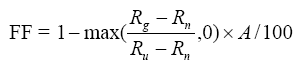,

where A = the area (m2) of currently connected roof to be drained by the tank; Rg = number of days of runoff per year from A following treatment by tank (or garden, see below); Rn = frequency of runoff from A in pre-urban state (12 days per year0; Ru = frequency of runoff from A before treatment (121 days per year). All three indices are standardized by impervious catchment area: one unit measures the environmental benefit from 100 m2.

The volume reduction target is based on the understanding that the replacement of vegetation by roofs or paving reduces the amount of rainwater that is lost back to the air through evapotranspiration. In the forest of Mt Evelyn before development, 85% of average annual rainfall was lost back to the air, and only 15% drained to the creek. The optimal target therefore is for 85% of rainfall to be used (in houses and exported to the sewerage treatment plant), or to be lost through vegetation.

The volume-reduction sub-index is calculated as: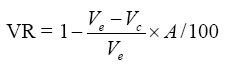,

where Ve = the excess volume of water generated by impervious surface A (i.e the difference between the volume of runoff from an area of forest equivalent to A and the volume of runoff from the impervious surface); Vc = the volume of water consumed from the tank (or lost from a rain-garden through evapo-transpiration: see below). One unit measures the environmental benefit from 100m2.

If a tank system uses all runoff that drains to it, it is possible for this subindex to be as high as 1.36 (in this region). We have chosen to encourage as much extraction as possible from tanks, because we know it will be impossible to lose the target volume of runoff from rain-gardens in this catchment.

The overall EB score for a tank = (0.25 x FF + 0.25 x VR). The maximum potential EB score for a 100 sq m impervious surface is 1. The maximum EB score a rainwater tank treating such a surface (without a leak for passive irrigation) can score is 0.59: [0.25* x 1 + 0.25 x 1.36]. If an appropriate slow leak is incorporated into the tank design to allow passive irrigation of an appropriately large area of garden, restoration of lost infiltration and evapotranspiration losses are achievable, meaning closer to the maximum potential score is possible.

EB calculator allows the modelling of four simple scenarios:
a) a tank by itself,
b) a rain-garden by itself
c) a tank and a rain-garden with no interaction (note in this case, an unchecked assumption is that diverted first flush water from the tank goes to the garden and not the stormwater drain), and
d) a tank whose overflow (and first flush) drains to the raingarden.

More complex scenarios can be modelled by using the R-functions outside the webpage environment, or by running EBI calculator in separate runs and doing some simple calculations. For instance, to estimate the EBI improvement of connecting an existing rainwater tank to household appliances, first calculate EBI scores for the existing set up, and then calculate the scores for the proposed set up. The EBI improvement that could attract a rebate is the difference between the two.

Raingarden behaviour is modelled using the function gardenmodel.

It uses hourly inflow data. If there is no tank upstream, the model uses hourly rainfall data from Croydon (1965, as above -other rainfall data can be used if gardenmodel is run using R: see above), converted to impervious runoff (by removing the first mm of rain in each day), and multiplied by the impervious catchment area to get inflow in litres per hour. If there is a tank upstream, then the daily overflow (and firstflush, if relevant) volume from the tank is converted to hourly data (with the overflow volume distributed among the hours to match the hourly runoff pattern). This calculation of inflows from a tank is done with with the functions 'distribute.firstflush','distribute.overflow', and 'compile.inflow'.

The function requires a filter profile as an input. The default filter profile for Mt Evelyn is as follows:
d.dm porosity Ksu.dm.h
2 1 0.25
5 0.4 0.25
5.05 0.4 0.00005
7.5 0.4 0.00005
12 0.6 0.00005
This default profile assumes a pond depth of 20cm (0.2dm, porosity of air = 1: this depth is subtracted from subsequent depths to calculate depth below the soil surface), and filter medium of loamy sand (to soil depth of 55cm (5.5 dm), porosity =0.4, with underlying gravel to 100cm deep (porosity = 0.6). It assumes that the top 30 cm (3 dm) has an infiltration capacity of 0.25 dm/h (25 mm/h), with the underlying soils with much lower infiltration capacity (0.005 mm/h). This soil profile is a close approximation to that found in our monitored raingarden.

These values are changed as a result of the pond and filter depths, depth of surface soil, and medium values that are entered on the web page. The depth of surface soil is zero for conventional rain-gardens, but a 60cm deep, sub-lawn gravel-filled infiltration system under 10 cm of surface soil would have depth of surface soil = 10cm). The soil profile for such a system would be adjusted to the following value:
d.dm porosity Ksu.dm.h
0 1 0.25
2 0.6 0.25
6 0.6 0.00005
The web page asks for dimensions of the rain-garden that allow it to adjust the above soil profiles, and to calculate the area and perimeter of the filter and pond. It also asks if the bottom is lined, and how much of the sides are lined. From this information, the gardenmodel works out the volume of water that can exfiltrate out through the sides or bottom of the garden at each time step given the depth of water in the garden. The web version assumes that the underlying infiltration rate is also 0.005 mm/h, but this can be altered using the argument Ksu.mm.h in the R version of the model.

The web page asks if the raingarden is vegetated (setting the isveg argument in the R model). If vegetated the model assumes that water is lost from the garden (when it contains any) at a rate equivalent to the potential evapotranspiration rate for Croydon. (Other transpiration rates could be incorporated by including in the rainfall input file.) If it is unvegetated no evapotranspiration is assumed. (Infiltration systems that are sunk below turf should be considered unvegetated as grass roots are unlikely to be deep enough to access water in the system). However, any infiltration system that is at laeast partly unlined is assumed to be accessible by trees within 3 m of the system. The calculator asks for the area (the web page asks for the diameter and converts to area) of the canopy of any nearby trees. The calculator assumes that nearby trees lose water to evapo-transpiration at 0.5 times the potential rate from their canopy area.

The critical variables in the gardenmodel function are Af (area of filter), Ap (area of pond), carea (impervious catchment area) all in sq m; Pf (perimeter of filter), Hf (height of the medium-filled filter), Hp (height of pond), Ho (Height of outlet pipe, if present), all in m (entered as cm on the web page). The web page assumes that an outlet pipe will be choked to permit an outflow at the rate of (impervious catchment area + filter area) * 0.1. This is approximately the highest rate of baseflow observed in Olinda Creek, and is therefore the highest permissible filtered flow. If the outlet pipe is a standard unchoked agricultural pipe, then outlet.rate.L.h should equal the infiltration rate of the filtration medium.

The gardenmodel steps through each time step, distributing existing water in the system plus inflow water between pond, filter, outlet pipe (if present), surrounding soils through exfiltration, and if the capacity of each of these sinks is exceeded by inputs, then water is allocated to overflow. Water quality of the filtered water flowing out of the system is modelled as follows.

Nitrogen concentration of stormwater inflows is assumed to be 2.2 mg/L. Overflow water is assumed to have the same nitrogen concentration as inflow water. and nitrogen removal is modelled according to the following table, and the resulting concentrations are assumed for water flowing out through the outflow pipe, as well as water exfiltrating into the surrounding soils (as it is assumed that N loss from soils is minimal, as it will be dominated by nitrate which is mobile through soils:
 Medium Vegetation type Normal (wet) After Dry Loamy sand Veg 59% If ADWP <=4 days then Removal = 59% Else Removal = 59% - 0.3% x ADWP (days) Unveg -101% If ADWP <=5 days then Removal = -101% Else Removal =-101% - 0.2% x ADWP (days) Sand Veg 72% 72% Unveg 32% 32% Gravel (Scoria) Unveg 39% 39%
Note the calculator does not allow the option of a vegetated gravel rain-garden.

Phosphorus concentration of inflow water is assumed to equal 0.35 mg/L. For outflow pipe water from sand or loamy sand systems, phosphorus concentration is assumed to be 26% of inflow concentration if vegetated, and 14% of inflow concentrations in unvegetated systems. Unvegetated gravel systems are assumed to reduce P concentrations by 20%. Water that exfiltrates into surrounding soils is assumed to lose almost all phosphorus, with a concentration of 0.001 mg/L assumed.

It is assumed that all sand or loamy sand systems are very efficient at reducing total suspended solids (99% removal through outlet pipes). Unvegetated gravel systems are assumed to reduce TSS concentrations by 20%.

The gardenmodel function produces a list of the following values:

1. budget, a list of vectors of hourly values for inflow (flowin), outflow through an outlet pipe if present (out), evapotranspiration loss (et), nitrogen load in outflow (Nout), nitrogen concentration of outflow (Nconcout), phosphorus concentration of outflow (Pconcout), total suspended solid concentration of outflow (TSSout), overflow (over), volume of water in pond (store.pond), volume of water in filter (store.filter), exfiltration flow to surrounding soils (Qexf), height of water in system (height.dm).
2. filter profile used.
The environmental benefit scores are calculated using the function garden.EBstats, which requires as input:
• budget, as above
• carea, impervious catchment area of direct runoff inputs into raingarden
• gardenarea, area of the filter
• mean.annrain.mm, mean annual rainfall, set to 950 mm for Mt Evelyn in the web page
• ndaysro.target, target number of days for overflow from the system, set to 12 for Mt Evelyn
• ndaysro.urban, number of days of impervious runoff = number of days with >1 mm rainfall, set to 121 for Mt Evelyn
• Nconc.target, target x percentile nitrogen concentration for any flow out of the system, set to 0.6 mg/L for Mt Evelyn
• Pconc.target, target x percentile phosphorus concentration for any flow out of the system, set to 0.05 mg/L for Mt Evelyn
• TSSconc.target, target x percentile nitrogen concentration for any flow out of the system, set to 20 mg/L for Mt Evelyn
• percentile, value of x in the above percentiles, set to 50, i.e. medians
• filtro.target, permissible flow rate out of the outflow pipe
The EB score of the garden is calculated based on the total area of all upstream impervious surfaces: those draining directly to the garden plus catchment areas of any tanks that drain into the garden. The EB score is the mean of four sub-indices
1. The flow-frequency sub-index, FF
2. The volume-reduction index, VR. Both of these indices were described for tanks, above.
3. The water-quality sub-index, WQ, calculated as the mean of the following three sub-sub-indices: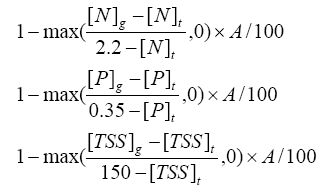,

where [N], [P], [TSS] are concentrations of nitrogen, phosphorus and total suspended solids, respectively. [X]g is the median (or other defined percentile) concentration of element X flowing from the system, and [X]t is the target concentration as defined above. The first number in each denominator is the assumed concentration of untreated stormwater in mg/L. A is the impervious catchment area, as defined above for the other sub-indices.
4. The filtered flow volume index, FV. calculated as: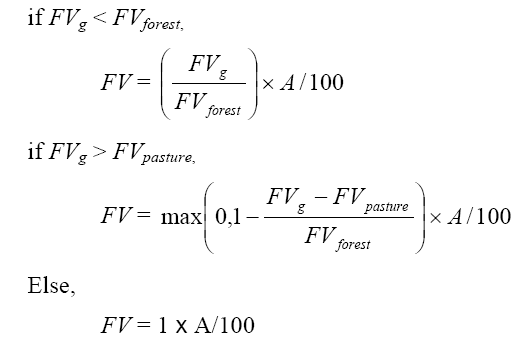,

where FVg = the volume of filtered water flowing out of the system, both through the outlet pipe (if present), and through exfiltration to the surrounding soils. FVforest and FVpasture represent the bounds of ideal filtered flow volume. They are derived from the model of Zhang et al. (2001) that predicted evapotranspiration and runoff from catchments with forest and pasture vegetation, as a function of annual rainfall (see figure below). Following the logic above for volume reduction, we assume the ideal volume of filtered flow corresponds to the volume of stream flow generated from a forest. However, we assume that good ecological health would still be feasible if stream flow volumes approached the volumes generated by grassed catchments. We therefore award systems a perfect score for the filtered volume index if the filtered volume is between the runoff volume from a forest and that from pasture.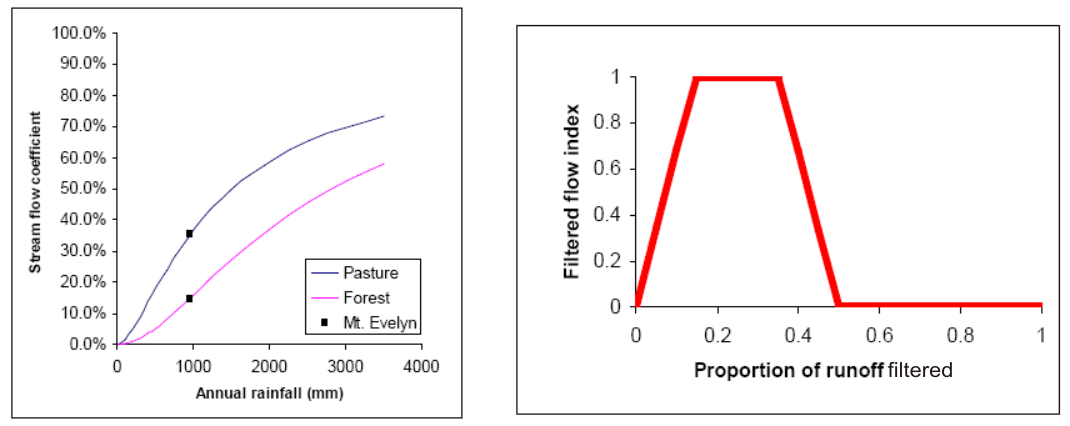The figure on the left shows the stream flow coefficients from forested and grassed (pasture) catchments as predicted by annual rainfall (Zhang et al. 2001). The black squares indicate the predicted stream flow coefficients for a catchment with Mt Evelyn's rainfall. The figure on the right shows the relationship between the filtered volume (as a proportion of total runoff) and FVg in Mt Evelyn.

Reference
Zhang L., Dawes W. R., and Walker G. R. (2001). Response of mean annual evapotranspiration to vegetation changes at catchment scale. Water Resources Research 37, 701-708.